## Chaos Ginger Bread Man Using MS Excel

Do you remember ginger bread man cookies? The purpose of this tutorial is to make fun using Mathematical Chaos Theory and MS Excel. I will guide you in a very gentle way to make Ginger bread man cards using Microsoft Excel.

Below are some examples of the cards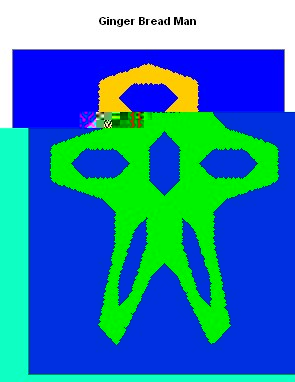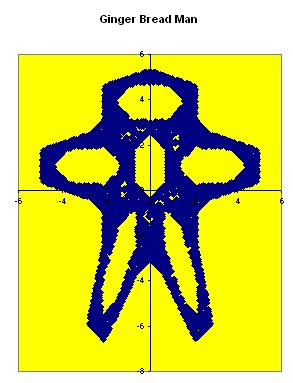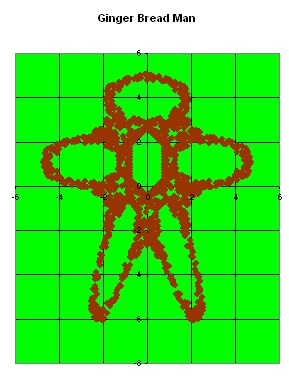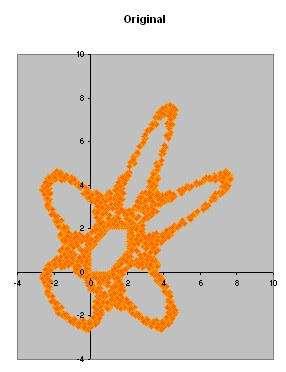The mathematics of Chaotic Ginger Bread man is very easy. You only need to use two formulas: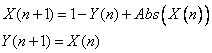The equation above is called difference equation or iterative map or dynamical system ( click here to learn more about it in my other tutorial ). If you never heard about it, do not be afraid with its name, you will be able to learn it very soon in this tutorial.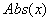is a function Absolute, sometimes written as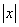to make all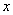into positive value.

To make plot of Ginger bread man, you need to put many points. Each point have coordinate name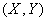. The interesting part is the relationship between one points coordinate to the other point. Each coordinate depend on the previous coordinate, in a certain manner that written in the equation above.

Let us call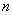to be the order of sequence that one point will influence the other point. We start with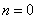and we assume the first point, say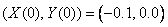. Then we apply the formula above to get the next points.

For example, for, we have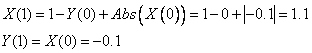For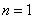, we have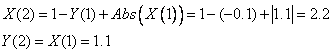The following table gives thecoordinate for the first eleven points

 n X Y 0 -0.1 0 1 1.1 -0.1 2 2.2 1.1 3 2.1 2.2 4 0.9 2.1 5 -0.2 0.9 6 0.3 -0.2 7 1.5 0.3 8 2.2 1.5 9 1.7 2.2 10 0.5 1.7

To make Ginger Bread Man Card, you need to compute thousand of points and plot those points. If you compute only a few hundred points, the results is look like the following picture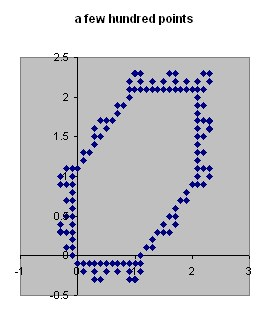If you plot thousand points, you will get similar to the card belowTo plot the graph, you use MS Excel Chart Wizard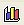and follow the wizard until Finish. Download the MS Excel companion of this tutorial here .